# Flat form

Jump to: navigation, search

A measurable-dimensional differential formon an open set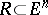such that: 1) the co-mass (cf. Mass and co-mass)for a given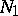; and 2) there exists anwithfor any simplex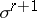satisfying the following condition: There exists a measurable,, such thatis measurable onand on any one of its boundaries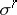, making up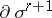; moreover,Here,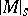denotes the-dimensional Lebesgue measure of the intersection of the setwith some-dimensional plane.

Ifis an-dimensional flat cochain in, there exists a bounded-dimensional form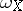inwhich is measurable in any simplexwith respect to the plane which contains, and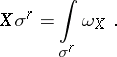(1)

Alsowhere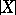is the co-mass of the cochain. Conversely, to any-dimensional flat forminthere corresponds, according to formula (1), a unique-dimensional flat cochain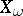for any simplexwhich satisfies the above condition; moreover,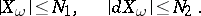The formand the cochainare called associated. Forms associated with the same cochain are equivalent, i.e. are equal almost-everywhere in, and comprise the flat representative.

There is a one-to-one correspondence between the-dimensional flat cochainsand the classes of equivalent bounded measurable functions, given by, and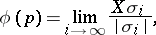where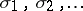is a sequence of-dimensional simplices contracting towards the pointsuch that their diameters tend to zero, but such that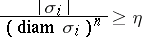for some value of, where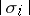is the volume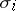for all.

Let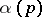be a measurable summable function inwhose values are-vectors; it is said to correspond to an-dimensional flat chain if(2)

for all-dimensional flat cochains(is then called a Lebesgue chain). The mapping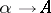is a linear one-to-one mapping of the set of equivalence classes of functionsinto the space of flat chains; also,, where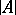is the mass of the chain, (cf. Mass and co-mass) and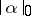is the mass of the-vector. In addition, the set of images of continuous functionsis dense in.

Formulas (1) and (2) generalize similar results for sharp forms and sharp cochains (cf. Sharp form); for instance, the differential of the flat form, defined by the formula, is also a flat form, and Stokes' theorem: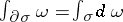is valid for any simplex; an-dimensional flat cochain is the weak limit of smooth cochains, i.e. cochains for which the associated formsare smooth, etc.